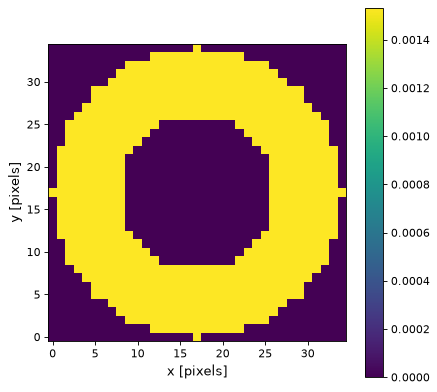# Ring2DKernel¶

class `astropy.convolution.``Ring2DKernel`(radius_in, width, **kwargs)[source]

2D Ring filter kernel.

The Ring filter kernel is the difference between two Tophat kernels of different width. This kernel is useful for, e.g., background estimation.

The generated kernel is normalized so that it integrates to 1.

Parameters

Inner radius of the ring kernel.

widthnumber

Width of the ring kernel.

mode`str`, optional
One of the following discretization modes:
• ‘center’ (default)

Discretize model by taking the value at the center of the bin.

• ‘linear_interp’

Discretize model by performing a bilinear interpolation between the values at the corners of the bin.

• ‘oversample’

Discretize model by taking the average on an oversampled grid.

• ‘integrate’

Discretize model by integrating the model over the bin.

factornumber, optional

Factor of oversampling. Default factor = 10.

Examples

Kernel response:

```import matplotlib.pyplot as plt
from astropy.convolution import Ring2DKernel
ring_2D_kernel = Ring2DKernel(9, 8)
plt.imshow(ring_2D_kernel, interpolation='none', origin='lower')
plt.xlabel('x [pixels]')
plt.ylabel('y [pixels]')
plt.colorbar()
plt.show()
```

(png, svg, pdf)